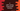# How to split a string in C using strtok library function## How to split a string in C using strtok library function:

strtok is defined in string.h header file. This is an important method and we can use it to split a string in C easily. e.g. if you have a list of comma separated words, we can use this method to split the string at comma and we can get all the words.

In this post, we will learn the definition of strtok and how to use it with examples.

## Definition of strtok:

strtok is defined as like below:

``char * strtok ( char * str, const char * del );``

str is the given string and del is a character array containing the delimeters.

It returns a pointer to the beginning of the token is returned if a token is found. Otherwise, a null pointer is returned.

## Example of strtok:

Let’s take a look at the below program:

``````#include <stdio.h>
#include <string.h>

int main()
{
char str[] = "one,two,three";
char delim[] = ",";

char *ptr = strtok(str, delim);

while (ptr != NULL)
{
printf("%s\n", ptr);
ptr = strtok(NULL, delim);
}
}``````

If you run it, it will print:

``````one
two
three``````### Example of strtok with multiple delimeters:

We can also use strtok with multiple delimeters as like below:

``````#include <stdio.h>
#include <string.h>

int main()
{
char str[] = "one,two-three:four+five";
char delim[] = ",-:+";

char *ptr = strtok(str, delim);

while (ptr != NULL)
{
printf("%s\n", ptr);
ptr = strtok(NULL, delim);
}
}``````

It will print:

``````one
two
three
four
five``````